A parabola that cuts the-axis at integer values ofwill have a quadratic expression that factors easily.  The factored form is useful for both graphing and for solving quadratic equations.

If a parabola does not cut theaxis at all, or if the-intercepts are irrational numbers, factoring the quadratic expression is unlikely to be a helpful process.

# Review

The general form for a quadratic expression isWhen the coefficient of,is 1 we have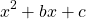. These expressions are the most straightforward to factor.

## Example 1:We need to find numbersandsuch thatA little thought or writing on paper leads us to the valuesandbecauseand.

Therefore we have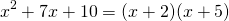Check this answer by expanding the brackets with FOIL or otherwise to confirm that the expression on the left hand side is equivalent to the expression on the right hand side.

## Example 2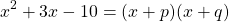We need to find numbersandsuch thatThe valuesandwork here because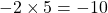and.

Therefore we have## Example 3: Difference of two squares

Check that this works by multiplying out the brackets:In generalOr, to be more general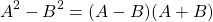Difference of two squares questions

1.2.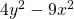3.# When the coefficient a is not 1: easy

These can also be quite straightforward if there turns out to be a common factor in all three terms, or if it can be understood as the difference between two squares, or if there is no constant term andis a common factor.

Example 4: No constant termThe highest common factor of these two terms is.Example 5: There is a common factor between all three termsFirst we notice that 2 divides all three terms. Let’s factor it out:Now, within the brackets, we have a trinomial withand so we simply find a factor pair that multiplies to 4 and adds to -5. I’m thinking -4 and -1 will do it!# When the coefficient a is not 1: not so easy

If none of these apply, the factoring can be quite tricky.

Here are four methods:

# Factoring by Limited Trial on Error

First, remember that factoring is about efficiency. If some time has been spent and no factors have been found, we may use an alternative technique (graphing, quadratic formula, completing the square…).

Here is how to do it by limited trial on error, that is, by testing the limited number of possibilities until a solution is found:

## Example 6:We need to examine the factor pairs of 3 and of 20:  (1,3) is the only factor pair of 3. Factor pairs of 20 include (1,20); (2,10); (4,5)

We can beginas (3,1) is the only factor pair of 3. Considering FOIL, the ‘F’ has now been dealt with. As we are only going to insert a factor pair of 20 the ‘L’ will also be dealt with.

We only need to consider the ‘O’ and the ‘I’. These must add to -11. We have three factor pairs to consider, knowing that one of the pairs must be negative to yieldas the last term.

Let’s try the factor pair (1,20) in all configurations:Let’s try the factor pair (2, 10) in all configurations but this time, just consider the O and the I terms, and we need not swap the signs as both givethe same value:Now the factor pair (4,5):Therefore.

Although this might seem tedious to go through the factor pairs, with a little practice one gets quite quick at checking the possibilities. If you have good multiplication table recall – or if you want to have better recall (use a printed multiplication table to help not a calculator), this is a good method for you.

# Practice to find zeros (roots)

As we saw on the page Factored form to graph, the factored form leads us quickly to the-intercepts of a parabola – if the-intercepts are integers or reasonably simple fractions. Use this applet to practice finding the roots of the parabola by factoring the quadratic expression.